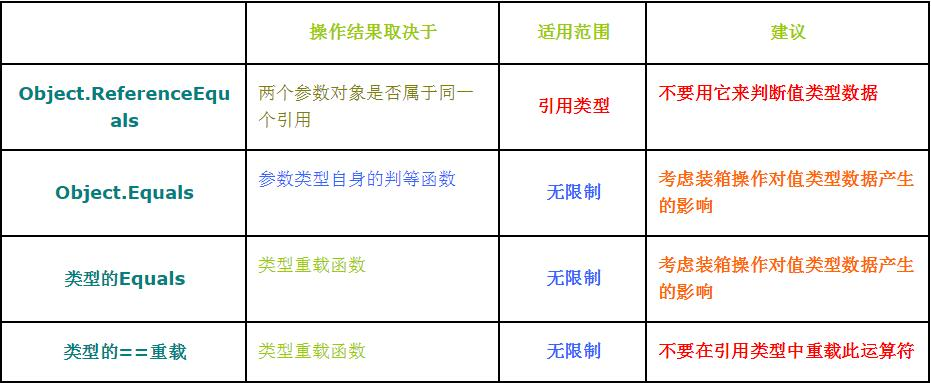# C# ==、Equals、ReferenceEquals 区别与联系

### 相关概念

.Net提供了ReferenceEquals、静态Equals，具体类型的Equals以及==操作符这四个判等函数。但是这四个函数之间有细微的关系，改变其中一个函数的实现会影响到其他函数的操作结果。

#### Object.ReferenceEquals这个静态函数，函数形势如下：

public static bool ReferenceEquals( object left, object right );

        A a2 = new A();
A a3 = new A();
Console.WriteLine(object.ReferenceEquals(a2, a3));//False
string a = "aasdsdsa";
string b = "aasdsdsa";
Console.WriteLine(object.ReferenceEquals(a, a));//True
Console.WriteLine(object.ReferenceEquals(a, b)); //True
int n = 10;
int m = 10;
Console.WriteLine(object.ReferenceEquals(m, m));//False
Console.WriteLine(object.ReferenceEquals(m, n)); //False
这是因为对于n这个数据装箱两次，而每次装箱后的地址有不同，而造成Object.ReferenceEquals( n, n )的结果永远为false。


#### Object.Equals这个静态函数，其形式如下：

public static bool Equals( object left, object right );

public static void Equals( object left, object right )
{
// Check object identity

if( left == right )
return true;
// both null references handled above

if( ( left == null ) || ( right == null ) )

return false;

return left.Equals( right );

}


            string a = "aasdsdsa";
string b = "aasdsdsa";
A a2 = new A();
A a3 = new A();
Console.WriteLine(object.ReferenceEquals(a2, a3));//False
Console.WriteLine(object.Equals(a2, a3));//;False
Console.WriteLine(object.Equals(a, a));//True
Console.WriteLine(object.Equals(a, b)); //True
int n = 10;
int m = 10;
Console.WriteLine(object.Equals(m, m));//True
Console.WriteLine(object.Equals(m, n)); //True

• 所谓自反，即a == a；

• 而对称，是a == b，则b == a；

• 传递是 a == b，b == c，则 a == c；

public override bool Equals( object right );

public class KeyData
{

private int nData;

public int Data

{

get{ return nData;}

set{ nData = value; }

}

public override bool Equals( object right )

{

//Check null

if( right == null )

return false;

//check reference equality

if( object.ReferenceEquals( this, right ) )

return true;

//check type

if( this.GetType() != right.GetType() )

return false;

//convert to current type

KeyData rightASKeyData = right as KeyData;

//check members value

}

}


if( this.GetType() != right.GetType() )

public static bool operator == ( KeyData left, KeyData right );

public struct KeyData

{

private int nData;

public int Data

{

get{ return nData;}

set{ nData = value; }

}

public static bool operator == ( KeyData left,  KeyData right )

{

return left.Data == right.Data;

}

public static bool operator != ( KeyData left, KeyData right )

{

return left.Data != right.Data;

}

}#### 那么在编写类型判等函数的时候，要注意些什么呢，给出如下几点建议。

• 要判断当前定义的类型是否具有判等的意义；

• 定义类型的判等函数要满足等价规则；

• 最后一点，值类型最好不要重载定义Equals函数，而引用类型最好不要重载定义==操作符。03-316253
10-152305
12-2839
08-2922
03-233195
10-07571
03-1523
05-29208
11-1545
08-26184
06-04461
11-2834
03-111624
07-2792
07-1246
07-051万+
03-29122
12-03129